# Uses of And function along with If function in Microsoft Excel 2010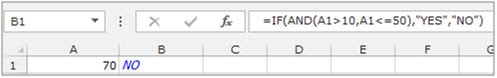In this article, we will learn to apply multiple conditions in a single formula using OR and AND function with the IF function.

Scenario and functions used

IF: -IF condition allows us to use multiple conditions in a cell. It helps to make the function flexible. Excel allows upto a max of 64 conditions which can be checked using this function.

Syntax of “IF” function:

 =IF(Logic_test, [Value_if True], [Value_if_False])

AND function works on logic_test. It helps you run multiple conditions in Excel. If every one of them is True, then only AND function returns True else False.

Syntax:

 =AND( Logic_test 1, [logic_test 2], ..)

Here the AND function returns True only if all the logical tests return True. And IF function then returns the value corresponding to the result obtained.

IF and AND Function syntax:

 IF(AND( Logic_test 1, [logic_test 2], ..),[Value_if True], [Value_if_False])

The IF and AND functions can be used together in many functions. However, before we proceed further, lets learn about the two functions individually and understand how they perform in Microsoft Excel 2010.

Example:

All of these might be confusing to understand. Let's understand how to use the function using an example. Here we will use IF with AND function in Excel. Let’s get this by an example here.

We have a list of Scores and We need to know under which criteria it occurs.Use the formula to match the criteria

Use the Formula:

 =IF(AND(B3 > 50, B3 < 60), "Below Average",IF(AND(B3 > 60, B3 < 90),"Average",IF(AND(B3 > 90,B3< 100),"Above Average","Top Score")))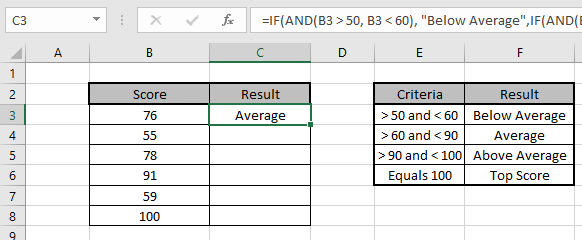Copy the formula in other cells, select the cells taking the first cell where the formula is already applied, use shortcut key Ctrl + D or dragging down from the right bottom of the selected cell.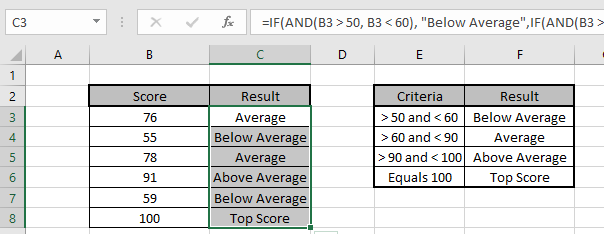We got the Results corresponding to the Scores.

You can use IF and AND function to meet multiple conditions in a single formula.

Example:Cell A1 and A2 contain the numbers 3 and 5 respectively. Lets put a condition that if the number in the cell is 3 then the formula should display “Yes” otherwise “No”.

=IF (A1=3,"Yes","No")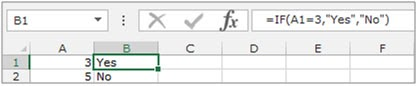AND: -Returns TRUE if all its arguments evaluate to TRUE; returns FALSE if one or more arguments evaluate to FALSE. It performs for all logical test.

Example:Column A contains 3 numbers and we need to check if the cell contains anumber greater than 10 and less than or equal to 50.

Syntax of “AND” function: =AND (logical1,[logical2]…)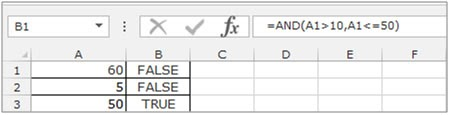Here we can see that the function returns False in 2 cells and True in 1 cell. Since both the conditions evaluated to False, the result was False. In the last row, both the conditions evaluated to True, hence the result is True.

Let’s take an example & understand how we can use the “And” function along with the “IF” function.

Our condition is - If A1 is greater than 10 and less than 50, return YES, else NO.

• Select the cell B1 and write the formula
• =IF(AND(A1>10,A1<=50),"YES","NO")
• Press enter on the keyboard.
• If the cell contains the number 60 then the function will return “NO” and if the number in the cell is greater than 10 and less than or equal to 50, then the function will return “YES”.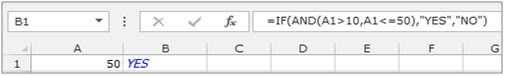So this is how we can use the IF and the AND formula together to arrive at the result based on two or more criteria.

Here are all the observational notes using the .... function in Excel
Notes :

1. Use the commas carefully, as commas ( , ) separates the one argument from another.
2. The function returns an error, then check the partial formula using the F9 key.

Hope this article about Uses of And function along with If function in Microsoft Excel 2010 is explanatory. Find more articles on logical formulas and related Excel formulas here. If you liked our blogs, share it with your friends on Facebook. And also you can follow us on Twitter and Facebook. We would love to hear from you, do let us know how we can improve, complement or innovate our work and make it better for you. Write to us at info@exceltip.com.

Related Articles :

IF with AND function : Implementation of logic IF function with AND function to extract results having criteria in Excel.

IF with OR function : Implementation of logic IF function with OR function to extract results having criteria in excel data.

How to use nested IF function : nested IF function operates on data having multiple criteria. The use of repeated IF function is nested IF excel formula.

SUMIFS using AND-OR logic : Get the sum of numbers having multiple criteria applied using logic AND-OR excel function.

Minimum value using IF function : Get the minimum value using the excel IF function and MIN function on array data.

How to use wildcards in excel : Count cells matching phrases using the wildcards in excel

Popular Articles :

How to use the IF Function in Excel : The IF statement in Excel checks the condition and returns a specific value if the condition is TRUE or returns another specific value if FALSE.

How to use the VLOOKUP Function in Excel : This is one of the most used and popular functions of excel that is used to lookup value from different ranges and sheets.

How to Use SUMIF Function in Excel : This is another dashboard essential function. This helps you sum up values on specific conditions.

How to use the COUNTIF Function in Excel : Count values with conditions using this amazing function. You don't need to filter your data to count specific values. Countif function is essential to prepare your dashboard.

Terms and Conditions of use

The applications/code on this site are distributed as is and without warranties or liability. In no event shall the owner of the copyrights, or the authors of the applications/code be liable for any loss of profit, any problems or any damage resulting from the use or evaluation of the applications/code.